Courses

# Basic & Electric Circuits - 2

## 20 Questions MCQ Test Topicwise Question Bank for Electrical Engineering | Basic & Electric Circuits - 2

Description
This mock test of Basic & Electric Circuits - 2 for Electrical Engineering (EE) helps you for every Electrical Engineering (EE) entrance exam. This contains 20 Multiple Choice Questions for Electrical Engineering (EE) Basic & Electric Circuits - 2 (mcq) to study with solutions a complete question bank. The solved questions answers in this Basic & Electric Circuits - 2 quiz give you a good mix of easy questions and tough questions. Electrical Engineering (EE) students definitely take this Basic & Electric Circuits - 2 exercise for a better result in the exam. You can find other Basic & Electric Circuits - 2 extra questions, long questions & short questions for Electrical Engineering (EE) on EduRev as well by searching above.
QUESTION: 1

### A current of 3 A flows through a resistor of 20 ohms. The energy dissipated in the resistor per minute is

Solution:

Power dissipated by the resistor is
P = I2 R = 32 x 20 = 180 W
Now, energy dissipated by the resistor in one hour (or 60 min)
= [180 x 60] watt-min
∴ Energy dissipated by the resistor in one minutes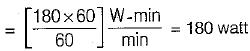QUESTION: 2

### A long uniform coil of a inductance L henries and associated resistance R ohms is physically cut into two exact halves which are then rewound in parallel. The resistance and inductance of the combination are

Solution:

We know that,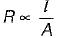and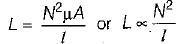when coil is cut into two equal halves,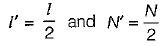(Area of cross - section = constant)
So,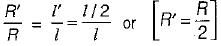Also,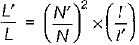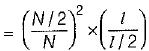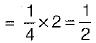So,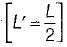Hence, new values of resistance and inductance which are reconnected in parallel is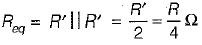and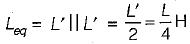QUESTION: 3

### In the circuit shown, the power dissipated in the resistor R is 1 W when only source ‘1’ is present and ‘2’ is replaced by short circuit. The power dissipated in the same resistor R is 4 W when only source '2’ is present and '1' is replaced by a short circuit When both the sources ‘1’ and ‘2’ are present, the power dissipated in R will be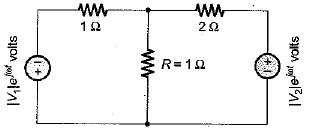Solution:

We have;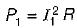or,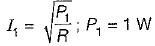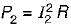or,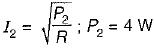When both the sources are present, net current through R will be
I = (I2 - I1
[as polarity of |V1| is reverse]
So, power loss in R is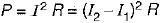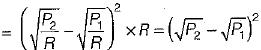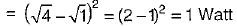QUESTION: 4

If v, w, q stand for voltage, energy and charge, then v can be expressed as:

Solution:
QUESTION: 5

In the figure shown below, φ = power-factor angle, W = watts, VA = volt ampere and VAr = volt-ampere .reactive for an ac circuit. The correct figure is

Solution: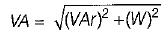QUESTION: 6

A circuit possesses resistance R and inductive reactance XL in series, its susceptance is given by

Solution:

Susceptance is the imaginary part of admittance,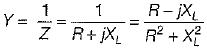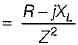or,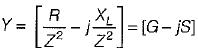Here, G = Conductance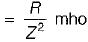and S = Susceptance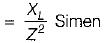QUESTION: 7

If V = a + jb and I = c + jd, then the power is given by

Solution:

P = VI* = (a + jb).(c + jd)*
= (a + jb) (c - jd)
= (ac + bd) + j(bc - ad)
= P + jQ
Here, P = Active power
and Q = Reactive power

QUESTION: 8

A 230 V, 100 W bulb has resistance RA and a 230 V, 200 W bulb has resistance RB. Here,
1. RA > RB
2. RB > RA
3. RA = 2 RB
4. RB = 2 RA
5. RA = 4 RB
From these, the correct answer is

Solution:

Power,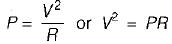Since voltage ratings of both bulbs are same, therefore
PR = constant
or, PA RA = PB RB
or, 100 x RA = 200 x RB
or, RA = 2 RB
Thus, RA > RB

QUESTION: 9

The voltage phapor of a circuit is 10∠15° V and the current phasor is 2∠- 45° A. The active and the reactive powers in the circuit are

Solution:

S = VI* = (10∠15°) (2∠ - 45°)*
= 20∠15 + 45° = 20∠60°
= 20 (cos 60° + j sin 60°)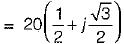= (10 + j10√3) = (10 + j17.32)
= P+ jQ

QUESTION: 10

In the circuit shown below, if the power consumed by the 5 Ω resistor is 10 W, then power factor or the circuit will be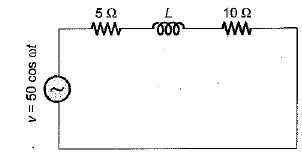Solution:

Given, power consumed is
P = IReq
or,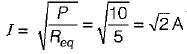Also,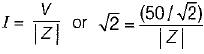or, |Z| = 25Ω
or,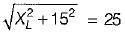or,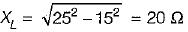Hence, p.f. of given circuit is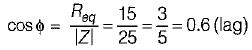QUESTION: 11

The minimum requirements for causing flow of current are.

Solution:
QUESTION: 12

Ohm’s law is applicable to

Solution:

Carbon resistor and semiconductors have nonlinear relationship between V and I. Hence, Ohm’s law is not applicable. Also, these are not bilateral.

QUESTION: 13

For a fixed supply voltage, the current flowing through a conductor will increase when its

Solution: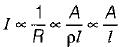When l is reduced. I will be increased and vice-versa.

QUESTION: 14

Two registors R1, and R2 give combined resistance of 4.5 Ω when in series and 1 Ω when in parallel. The resistances are

Solution:

R1 + R2 = 4.5 ...(i)
and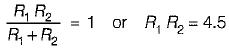∴ (R1-R2)2 = (R1 + R2)- 4R1R2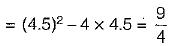or,...(ii)
On solving equations (i) and (ii), we get
R1 = 3 Ω and R2 = 1.5 Ω
or, R1 = 1.5 Ω and R2 = 3Ω.

QUESTION: 15

Which of the following is not equivalent to watts?

Solution:
QUESTION: 16

Two heaters, rated at 1000 W, 250 V each are connected in series across a 250 V, 50 Hz ac mains. The total power drawn from the supply would be

Solution:

For series connection,
Req = R1 + R2
or,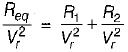or,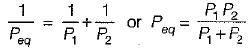Given,
P1 = P= 1000 W
∴ Peq = 500 Watt

QUESTION: 17

A 100 watt light bulb burns on an average of 10 hours a day for one week. The weekly consumption of energy will be

Solution:

Energy consumption per week is
W= 100 x 10 x 7
= 7000 kW - hr
= 7 units

QUESTION: 18

Assertion (A): The direction of flow of conventional current is taken opposite to that of electrons.
Reason (R): Electrons have negative charge.

Solution:
QUESTION: 19

Match List-I (Materials) with List-lI (Range of resistivity) and select the correct answer using the codes given below the lists:
List-I
A. Conducting materials
B. Semiconductor materials
C. insulating material
List-II
1. 100 to 102 Ω-m
2. 10-8 to 10-6 Ω-m
3. 1012to 1018 Ω-m
4. 1020 to 1030 Ω-m
Codes: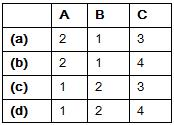Solution:
QUESTION: 20

A constant current source supplies a current of 200 mA to a load of 2 kΩ When the load is changed to 100 Ω, the load current is

Solution:

For a constant C.S., current will remain constant for all values of loads.